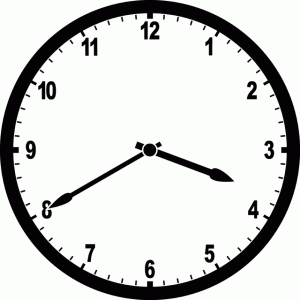# Coding Interview Question: Clock Angle## Question

Given two integers, an hour and a minute, write a function to calculate the angle between the two hands on a clock representing that time.

eg.`clockAngle(3:40) = 130°`

Once you think that you’ve solved the problem, click below to see the solution.

As always, remember that practicing coding interview questions is as much about how you practice as the question itself. Make sure that you give the question a solid go before skipping to the solution. Ideally if you have time, write out the solution first by hand and then only type it into your computer to verify your work once you've verified it manually. To learn more about how to practice, check out this blog post.

## Solution

How was that problem? You can check out the solution in the video below.

Here is the source code for the solution shown in the video: